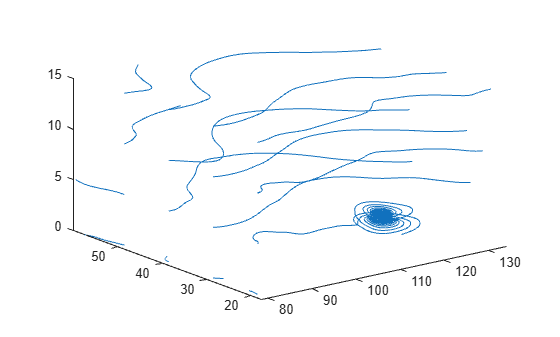# streamline

Plot streamlines from 2-D or 3-D vector data

•## Syntax

``streamline(X,Y,Z,U,V,W,startx,starty,startz)``
``streamline(U,V,W,startx,starty,startz)``
``streamline(X,Y,U,V,startx,starty)``
``streamline(U,V,startx,starty)``
``streamline(verts)``
``streamline(___,options)``
``streamline(ax,___)``
``lineobj = streamline(___)``

## Description

example

````streamline(X,Y,Z,U,V,W,startx,starty,startz)` draws streamlines from 3-D vector data `U`, `V`, `W`. Specify `X`, `Y`, and `Z` as the coordinate data. Specify `startx`,`starty`, and `startz` as the starting positions of the streamlines.```
````streamline(U,V,W,startx,starty,startz)` uses the default coordinate data for `U`, `V`, and `W`. The (x,y,z) location for each element in `U`, `V`, and `W` is based on the column, row, and page index, respectively.```

example

````streamline(X,Y,U,V,startx,starty)` draws streamlines from 2-D vector data `U` and `V`. Specify `X` and `Y` as the coordinate data. Specify `startx` and `starty` as the starting positions of the streamlines. ```
````streamline(U,V,startx,starty)` uses the default coordinate data for  `U` and `V`. The (x,y) location for each element in `U` and `V` is based on the column and row index, respectively. ```

example

````streamline(verts)` draws streamlines from vertices, specified as a cell array of vertex arrays (as returned by `stream2`, `stream3`, or `streamslice`).```
````streamline(___,options)` draws streamlines using the specified options, defined as a one- or two-element vector with the form `[step]` or `[step, maxvert]`, where `step` is the step-size for interpolating the vector data and `maxvert` is the maximum number of vertices in a streamline. Use this option with any of the input arguments from the previous syntaxes. ```
````streamline(ax,___)` plots into the specified axes, instead of into the current axes object (`gca`). ```

example

````lineobj = streamline(___)` returns a `Line` object. Use `lineobj` to modify properties of the streamlines after creating them. For a list of properties, see Line Properties.```

## Examples

collapse all

Load the `wind` dataset, which loads the 3-D arrays `u`, `v`, `w`, `x`, `y`, and `z`, representing air currents over regions of North America. Plot streamlines that start at evenly spaced points on the plane `x = 80`.

```load wind [startx,starty,startz] = meshgrid(80,20:10:50,0:5:15); streamline(x,y,z,u,v,w,startx,starty,startz) axis tight view(3);```Define arrays `x`, `y`, `u`, and `v`. Create a quiver plot to visualize the vector data. Then, plot streamlines that start at different points along the line `y = 1`.

```[x,y] = meshgrid(0:0.1:1); u = x; v = -y; quiver(x,y,u,v) startx = 0.1:0.1:1; starty = ones(size(startx)); streamline(x,y,u,v,startx,starty)```Load the `wind` dataset and use `stream3` to compute the vertices of streamlines that start at evenly spaced points on the plane `x = 80`. Then, plot the streamlines from the vertex data.

```load wind [startx,starty,startz] = meshgrid(80,20:10:50,0:5:15); verts = stream3(x,y,z,u,v,w,startx,starty,startz); streamline(verts) axis tight view(3);```Plot streamlines and return the line objects in `l`.

```[x,y] = meshgrid(-10:10); u = 2.*x.*y; v = y.^2 - x.^2; l = streamslice(x,y,u,v); axis tight```Set the line width of the streamlines to 2. Set their color to red. Use the `set` function to change the properties of all the lines at the same time.

```set(l,'LineWidth',2) set(l,'Color','r');```## Input Arguments

collapse all

x-axis coordinate data, specified as a 2-D or 3-D array. It must be monotonic, but does not need to be uniformly spaced.

`X`, `Y`, `Z`, `U`, `V`, and `W` must be the same size.

You can use the `meshgrid` function to create `X`, `Y`, and `Z` matrices.

y-axis coordinate data, specified as a 2-D or 3-D array. It must be monotonic, but does not need to be uniformly spaced.

`X`, `Y`, `Z`, `U`, `V`, and `W` must be the same size.

You can use the `meshgrid` function to create `X`, `Y`, and `Z` matrices.

z-axis coordinate data, specified as a 3-D array. It must be monotonic, but does not need to be uniformly spaced.

`X`, `Y`, `Z`, `U`, `V`, and `W` must be the same size.

You can use the `meshgrid` function to create `X`, `Y`, and `Z` matrices.

x-components of vector data, specified as a 2-D or 3-D array.

`X`, `Y`, `Z`, `U`, `V`, and `W` must be the same size.

y-components of vector data, specified as a 2-D or 3-D array.

`X`, `Y`, `Z`, `U`, `V`, and `W` must be the same size.

z-components of vector data, specified as a 3-D array.

`X`, `Y`, `Z`, `U`, `V`, and `W` must be the same size.

x-axis streamline starting positions, specified as a vector or matrix.

`startx`, `starty`, and `startz` must be the same size.

y-axis streamline starting positions, specified as a vector or matrix.

`startx`, `starty`, and `startz` must be the same size.

z-axis streamline starting positions, specified as a vector or matrix.

`startx`, `starty`, and `startz` must be the same size.

Streamline vertices, specified as a cell array (as returned by `stream2`, `stream3`, or `streamslice`). Each element of the cell array is a matrix of vertices for one line.

Streamline drawing options, specified as a one- or two-element vector with the following form:

• `[step]`

• `[step,maxvert]`

Where `step` is the step-size used for interpolating the vector data and `maxvert` is the maximum number of vertices in a streamline.

The default step-size is `.1` and the default maximum number of vertices is `1000`.

Target axes, specified as an `Axes` object. If you do not specify the axes, then the `streamline` function uses the current axes.